Скачать презентацию Course 2 D 1453 2006 -07 Advanced Formal

e63db664e495399712282f994d8b952e.ppt

• Количество слайдов: 19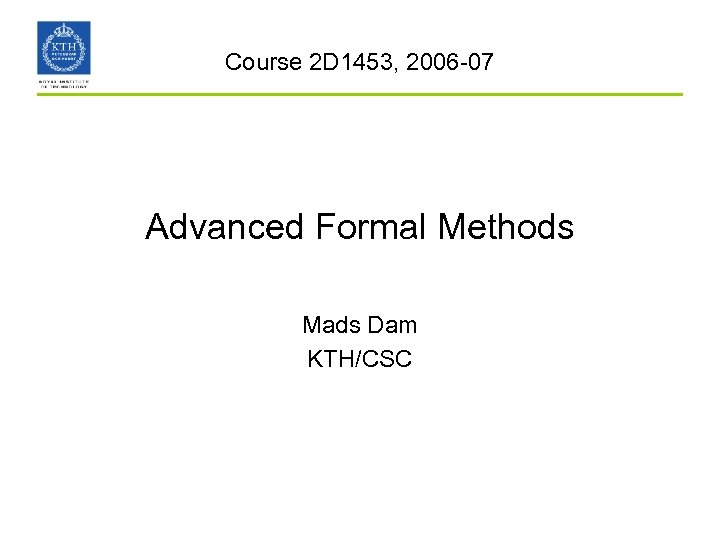Course 2 D 1453, 2006 -07 Advanced Formal Methods Mads Dam KTH/CSC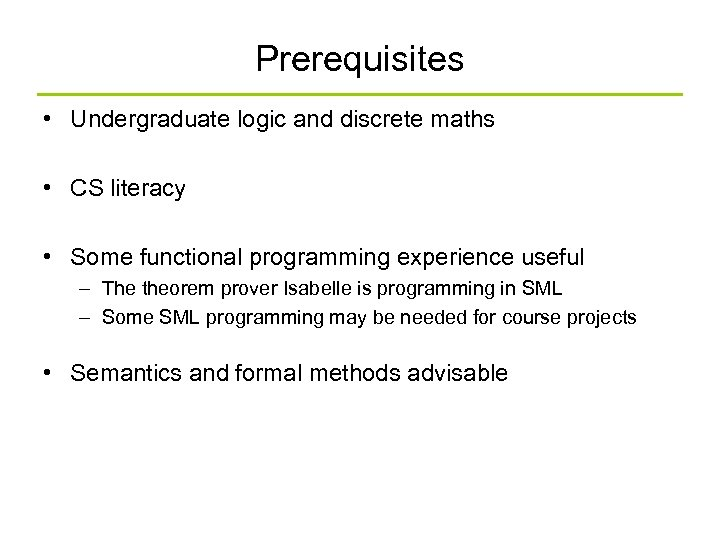Prerequisites • Undergraduate logic and discrete maths • CS literacy • Some functional programming experience useful – The theorem prover Isabelle is programming in SML – Some SML programming may be needed for course projects • Semantics and formal methods advisable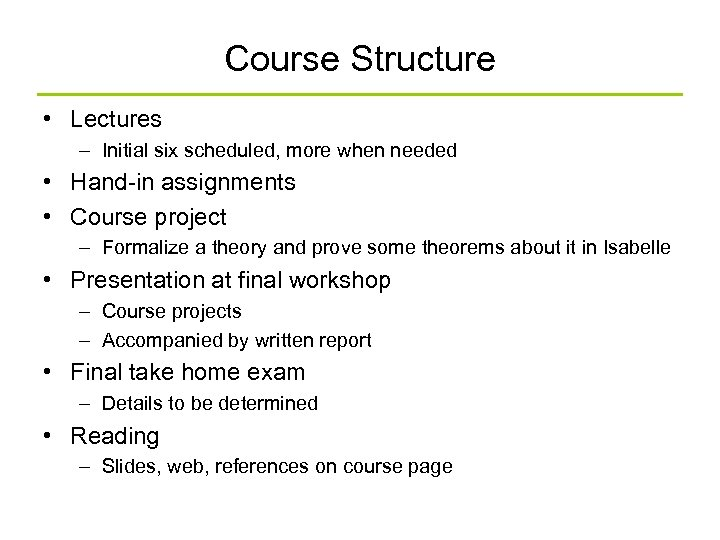Course Structure • Lectures – Initial six scheduled, more when needed • Hand-in assignments • Course project – Formalize a theory and prove some theorems about it in Isabelle • Presentation at final workshop – Course projects – Accompanied by written report • Final take home exam – Details to be determined • Reading – Slides, web, references on course page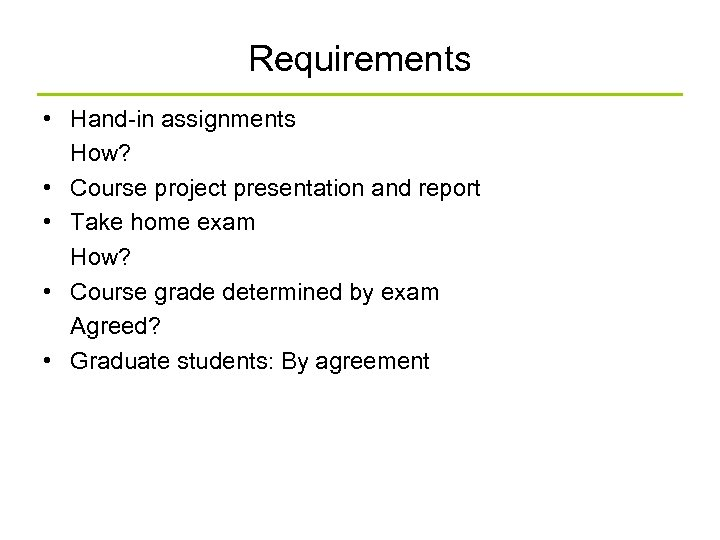Requirements • Hand-in assignments How? • Course project presentation and report • Take home exam How? • Course grade determined by exam Agreed? • Graduate students: By agreement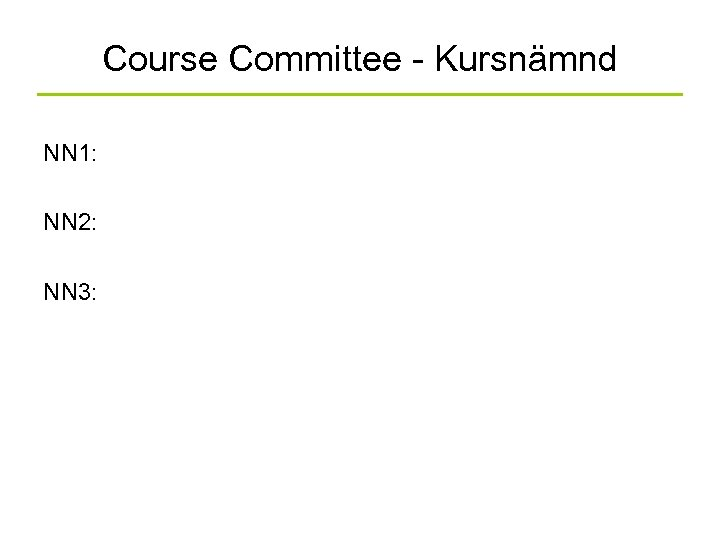Course Committee - Kursnämnd NN 1: NN 2: NN 3: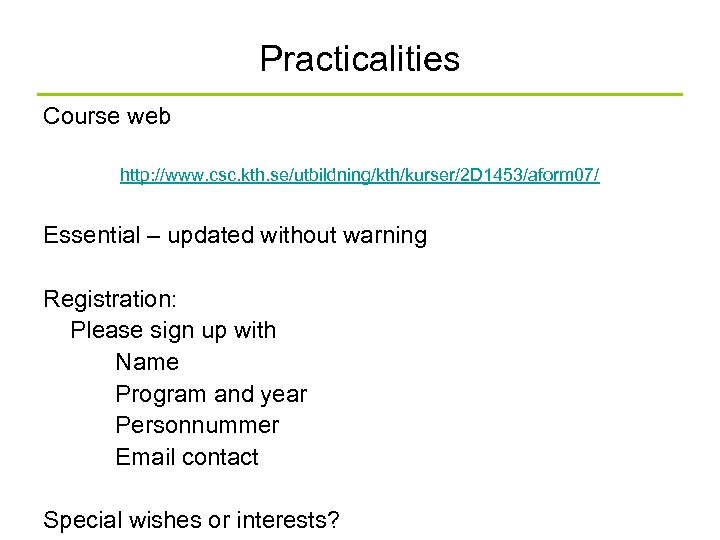Practicalities Course web http: //www. csc. kth. se/utbildning/kth/kurser/2 D 1453/aform 07/ Essential – updated without warning Registration: Please sign up with Name Program and year Personnummer Email contact Special wishes or interests?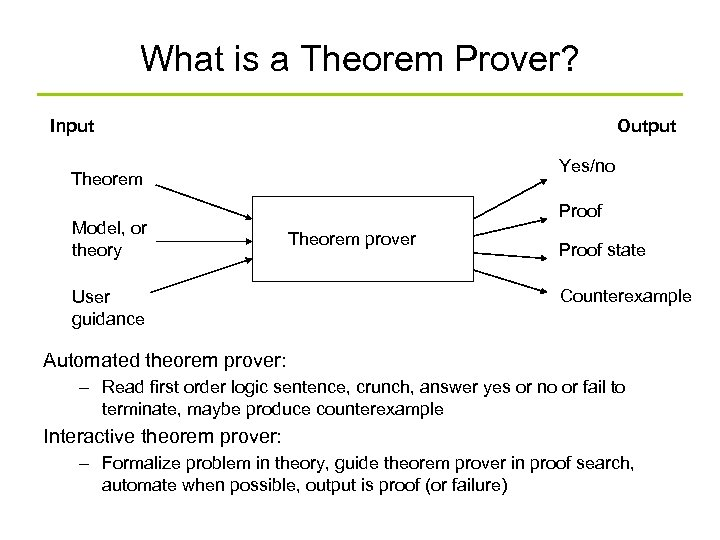What is a Theorem Prover? Input Output Yes/no Theorem Model, or theory User guidance Proof Theorem prover Proof state Counterexample Automated theorem prover: – Read first order logic sentence, crunch, answer yes or no or fail to terminate, maybe produce counterexample Interactive theorem prover: – Formalize problem in theory, guide theorem prover in proof search, automate when possible, output is proof (or failure)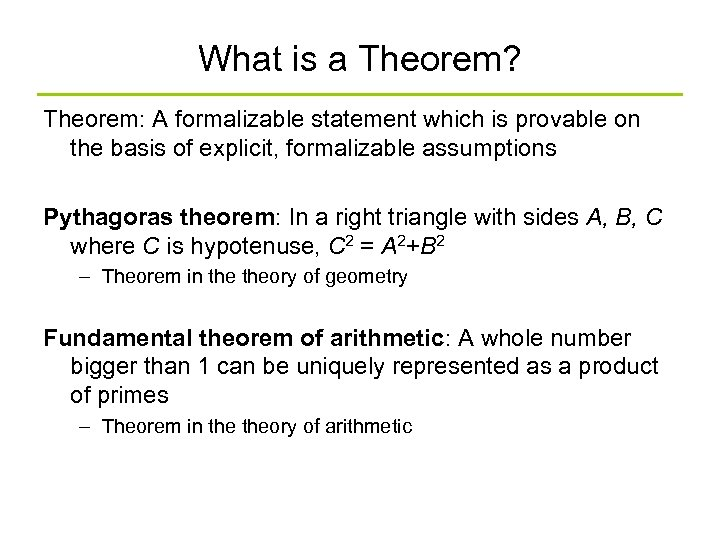What is a Theorem? Theorem: A formalizable statement which is provable on the basis of explicit, formalizable assumptions Pythagoras theorem: In a right triangle with sides A, B, C where C is hypotenuse, C 2 = A 2+B 2 – Theorem in theory of geometry Fundamental theorem of arithmetic: A whole number bigger than 1 can be uniquely represented as a product of primes – Theorem in theory of arithmetic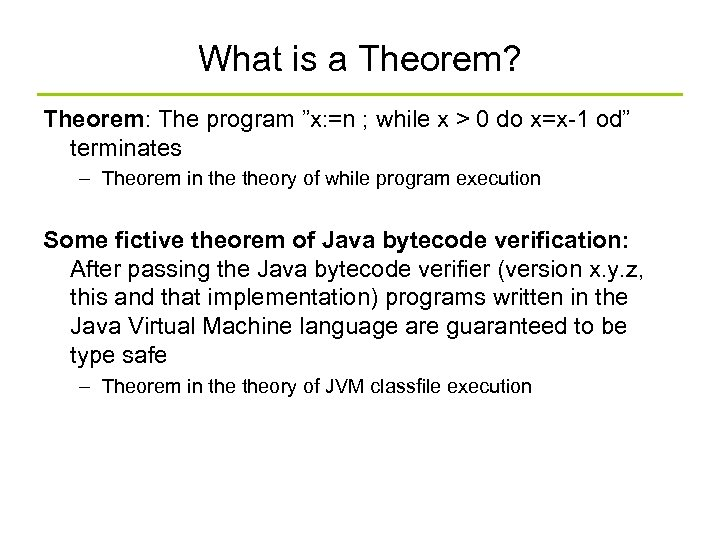What is a Theorem? Theorem: The program ”x: =n ; while x > 0 do x=x-1 od” terminates – Theorem in theory of while program execution Some fictive theorem of Java bytecode verification: After passing the Java bytecode verifier (version x. y. z, this and that implementation) programs written in the Java Virtual Machine language are guaranteed to be type safe – Theorem in theory of JVM classfile execution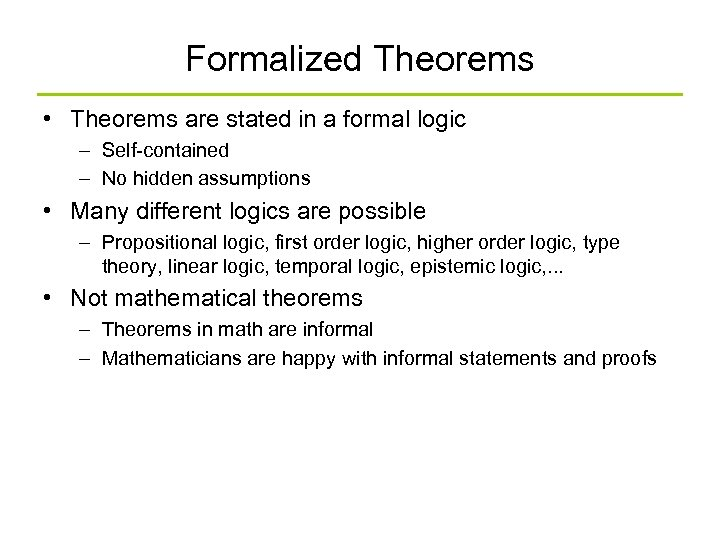Formalized Theorems • Theorems are stated in a formal logic – Self-contained – No hidden assumptions • Many different logics are possible – Propositional logic, first order logic, higher order logic, type theory, linear logic, temporal logic, epistemic logic, . . . • Not mathematical theorems – Theorems in math are informal – Mathematicians are happy with informal statements and proofs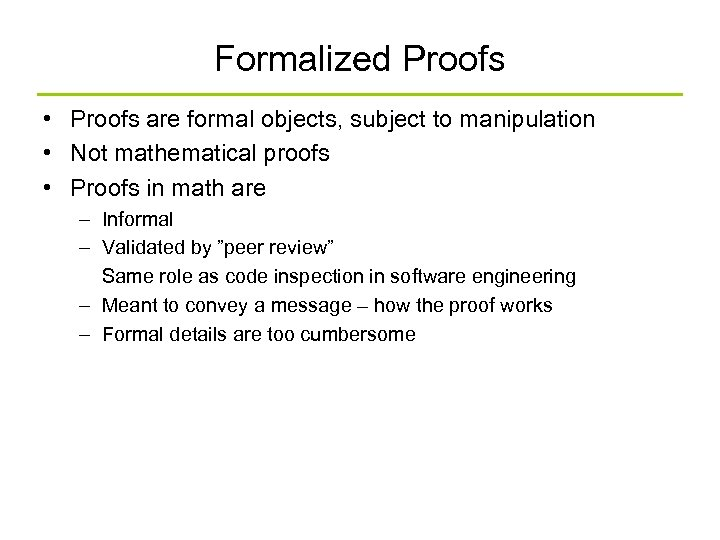Formalized Proofs • Proofs are formal objects, subject to manipulation • Not mathematical proofs • Proofs in math are – Informal – Validated by ”peer review” Same role as code inspection in software engineering – Meant to convey a message – how the proof works – Formal details are too cumbersome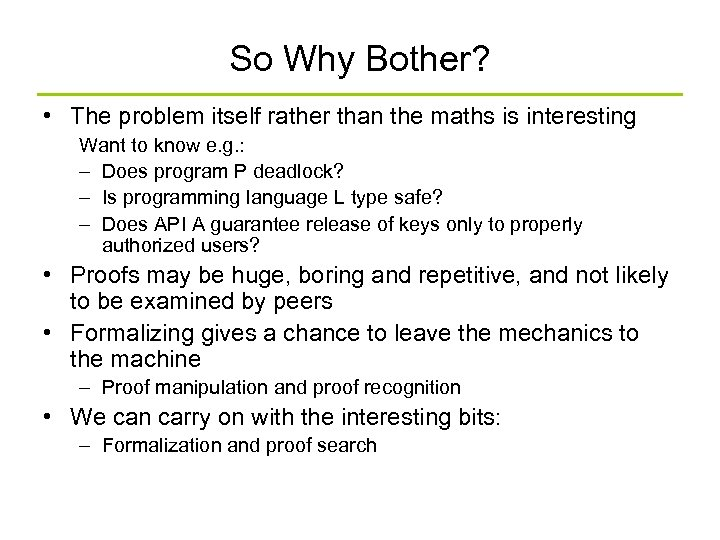So Why Bother? • The problem itself rather than the maths is interesting Want to know e. g. : – Does program P deadlock? – Is programming language L type safe? – Does API A guarantee release of keys only to properly authorized users? • Proofs may be huge, boring and repetitive, and not likely to be examined by peers • Formalizing gives a chance to leave the mechanics to the machine – Proof manipulation and proof recognition • We can carry on with the interesting bits: – Formalization and proof search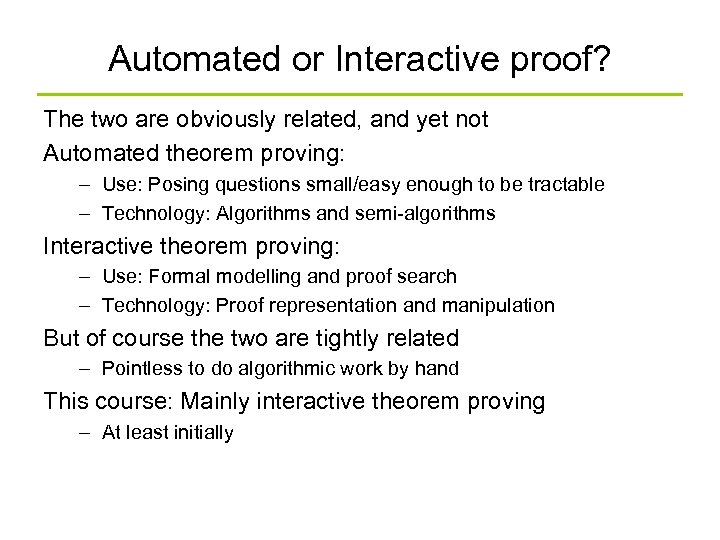Automated or Interactive proof? The two are obviously related, and yet not Automated theorem proving: – Use: Posing questions small/easy enough to be tractable – Technology: Algorithms and semi-algorithms Interactive theorem proving: – Use: Formal modelling and proof search – Technology: Proof representation and manipulation But of course the two are tightly related – Pointless to do algorithmic work by hand This course: Mainly interactive theorem proving – At least initially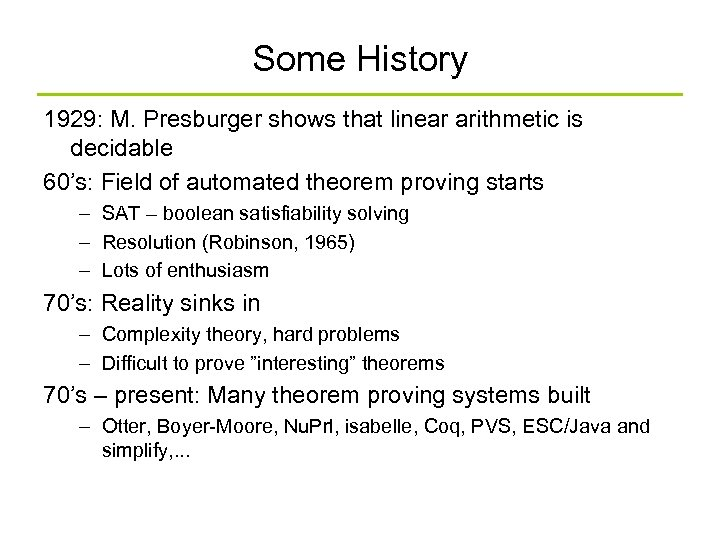Some History 1929: M. Presburger shows that linear arithmetic is decidable 60’s: Field of automated theorem proving starts – SAT – boolean satisfiability solving – Resolution (Robinson, 1965) – Lots of enthusiasm 70’s: Reality sinks in – Complexity theory, hard problems – Difficult to prove ”interesting” theorems 70’s – present: Many theorem proving systems built – Otter, Boyer-Moore, Nu. Prl, isabelle, Coq, PVS, ESC/Java and simplify, . . .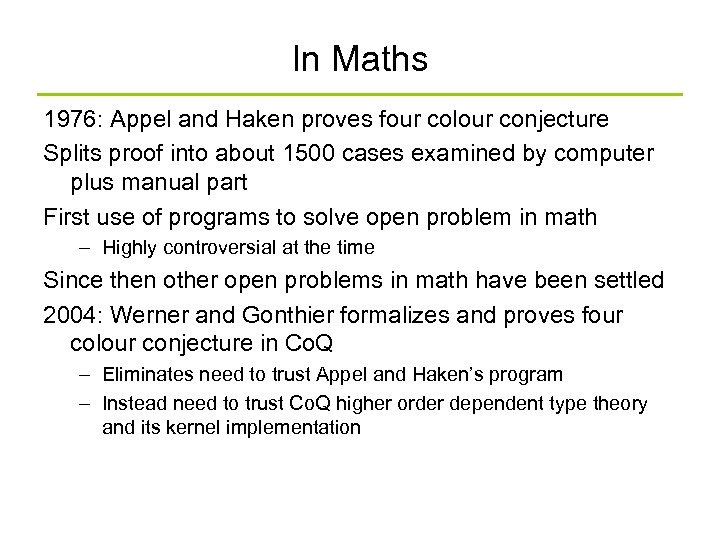In Maths 1976: Appel and Haken proves four colour conjecture Splits proof into about 1500 cases examined by computer plus manual part First use of programs to solve open problem in math – Highly controversial at the time Since then other open problems in math have been settled 2004: Werner and Gonthier formalizes and proves four colour conjecture in Co. Q – Eliminates need to trust Appel and Haken’s program – Instead need to trust Co. Q higher order dependent type theory and its kernel implementation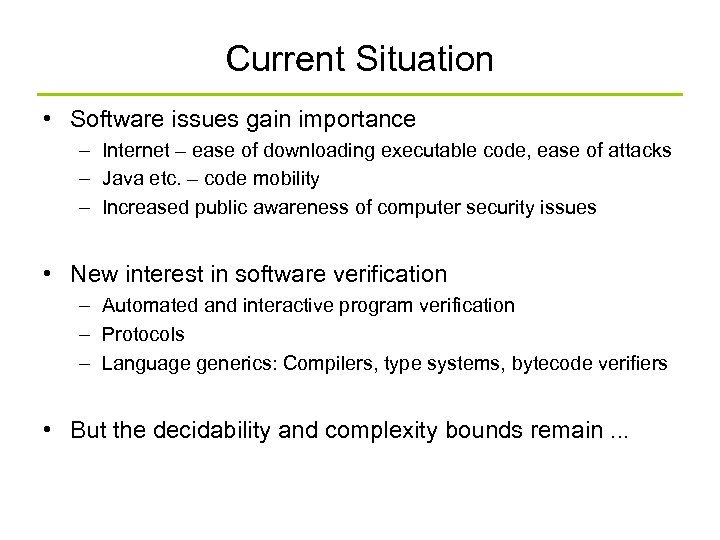Current Situation • Software issues gain importance – Internet – ease of downloading executable code, ease of attacks – Java etc. – code mobility – Increased public awareness of computer security issues • New interest in software verification – Automated and interactive program verification – Protocols – Language generics: Compilers, type systems, bytecode verifiers • But the decidability and complexity bounds remain. . .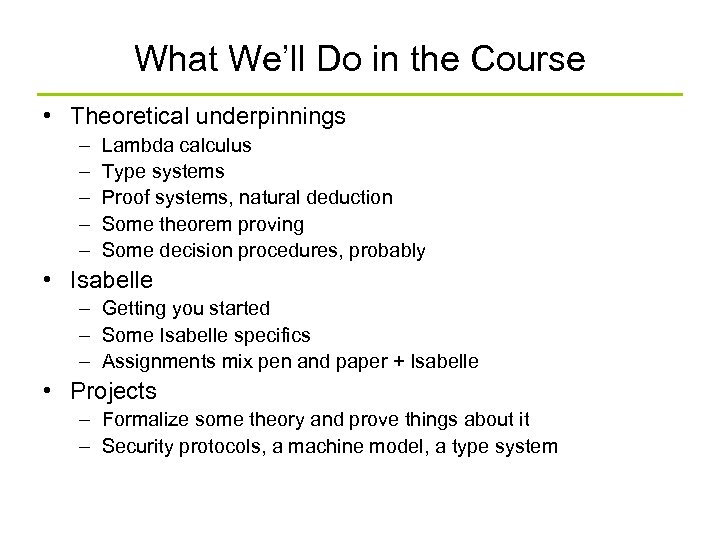What We’ll Do in the Course • Theoretical underpinnings – – – Lambda calculus Type systems Proof systems, natural deduction Some theorem proving Some decision procedures, probably • Isabelle – Getting you started – Some Isabelle specifics – Assignments mix pen and paper + Isabelle • Projects – Formalize some theory and prove things about it – Security protocols, a machine model, a type system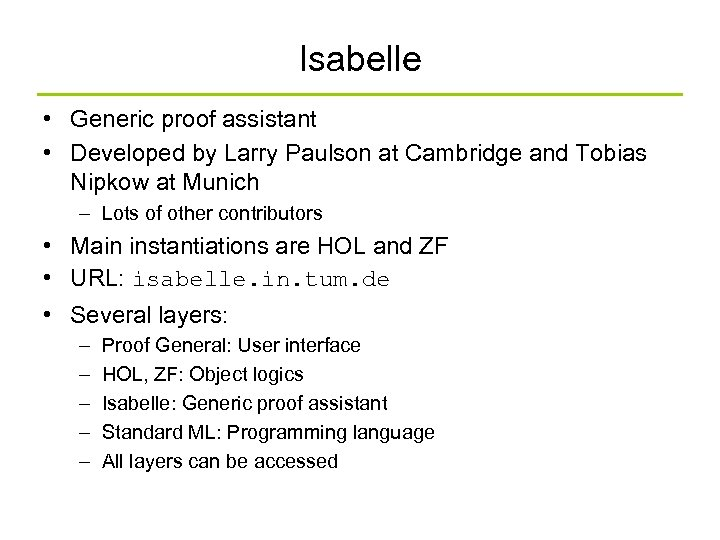Isabelle • Generic proof assistant • Developed by Larry Paulson at Cambridge and Tobias Nipkow at Munich – Lots of other contributors • Main instantiations are HOL and ZF • URL: isabelle. in. tum. de • Several layers: – – – Proof General: User interface HOL, ZF: Object logics Isabelle: Generic proof assistant Standard ML: Programming language All layers can be accessed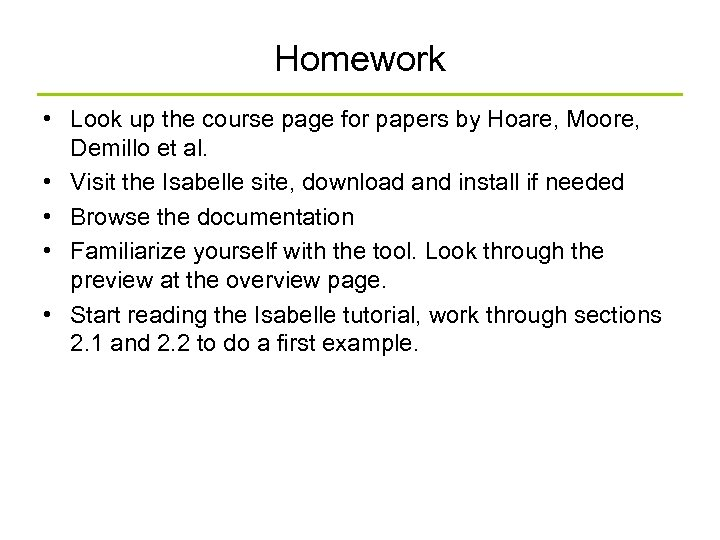Homework • Look up the course page for papers by Hoare, Moore, Demillo et al. • Visit the Isabelle site, download and install if needed • Browse the documentation • Familiarize yourself with the tool. Look through the preview at the overview page. • Start reading the Isabelle tutorial, work through sections 2. 1 and 2. 2 to do a first example.